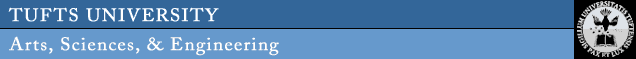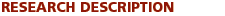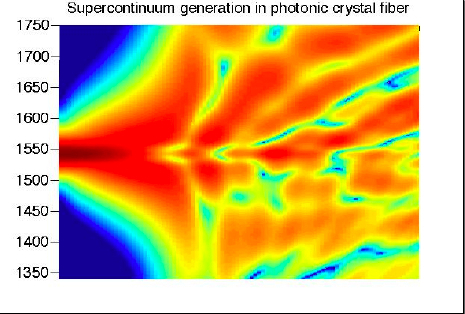JAMISON MOESER

 My work focuses on the application of techniques from nonlinear analysis to understand the behavior of evolution equations, particularly those arising in optics and biology.  The mathematical core of this work is the development of asymptotic theories, examination of well posedness, and demonstration of the existence and stability of solitary wave solutions for the modeling equations.I am currently working in the following areas Nonlinear PDEs and analysis One focus of my research is the qualitative behavior of solutions of nonlinear partial differential equations, including issues of well posedness and existence and stability of equilibrium solutions.   I am interested in the application of techniques from mathematical analysis, including dispersive estimates and calculus of variations, to help elucidate the behavior of physical systems.  Asymptotic and Numerical Approximations  Many important physical systems exhibit phenomena that evolve on multiple scales, and asymptotic and numerical methods are indispensable tools for studying such systems.  A particular area of interest to me is the development of averaging theories for both deterministic and stochastic PDEs.  Waves in inhomogenous media, including random media Much of my work has focused on the study of optical pulse propagation in spatially periodic or random media.  I am particularly interested in the interplay between nonlinearity and randomness.  Applications to optics and biology An important goal of my research is the integration of information from experiments, physical modeling, simulation, and mathematical analysis to gain insight into problems of significance in the physical world. The applications have ranged from pulse propagation in inhomogenous optical fibers, slab waveguides, and discrete waveguide arrays to supercontinuum generation in photonic crystal fibers and chemical reaction kinetics for signal transduction pathways.JAMISON MOESER Postdoctoral Associate Biomedical Engineering Tufts University E-mail: jamison.moeser@tufts.edu